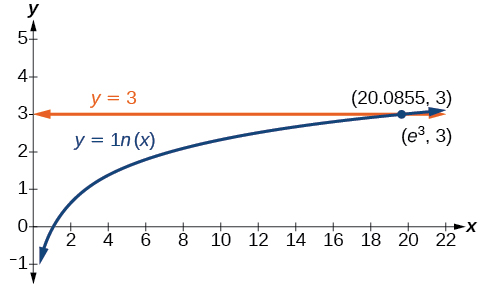# 6.6 Exponential and logarithmic equations  (Page 4/8)

 Page 4 / 8

## Using the definition of a logarithm to solve logarithmic equations

For any algebraic expression $\text{\hspace{0.17em}}S\text{\hspace{0.17em}}$ and real numbers $\text{\hspace{0.17em}}b\text{\hspace{0.17em}}$ and $\text{\hspace{0.17em}}c,$ where

## Using algebra to solve a logarithmic equation

Solve $\text{\hspace{0.17em}}2\mathrm{ln}x+3=7.$

Solve $\text{\hspace{0.17em}}6+\mathrm{ln}x=10.$

$x={e}^{4}$

## Using algebra before and after using the definition of the natural logarithm

Solve $\text{\hspace{0.17em}}2\mathrm{ln}\left(6x\right)=7.$

Solve $\text{\hspace{0.17em}}2\mathrm{ln}\left(x+1\right)=10.$

$x={e}^{5}-1$

## Using a graph to understand the solution to a logarithmic equation

Solve $\text{\hspace{0.17em}}\mathrm{ln}x=3.$

[link] represents the graph of the equation. On the graph, the x -coordinate of the point at which the two graphs intersect is close to 20. In other words $\text{\hspace{0.17em}}{e}^{3}\approx 20.\text{\hspace{0.17em}}$ A calculator gives a better approximation: $\text{\hspace{0.17em}}{e}^{3}\approx 20.0855.$The graphs of   y = ln x   and   y = 3   cross at the point   (e 3 , 3 ) , which is approximately (20.0855, 3).

Use a graphing calculator to estimate the approximate solution to the logarithmic equation $\text{\hspace{0.17em}}{2}^{x}=1000\text{\hspace{0.17em}}$ to 2 decimal places.

$x\approx 9.97$

## Using the one-to-one property of logarithms to solve logarithmic equations

As with exponential equations, we can use the one-to-one property to solve logarithmic equations. The one-to-one property of logarithmic functions tells us that, for any real numbers $\text{\hspace{0.17em}}x>0,$ $S>0,$ $T>0\text{\hspace{0.17em}}$ and any positive real number $\text{\hspace{0.17em}}b,$ where $\text{\hspace{0.17em}}b\ne 1,$

For example,

So, if $\text{\hspace{0.17em}}x-1=8,$ then we can solve for $\text{\hspace{0.17em}}x,$ and we get $\text{\hspace{0.17em}}x=9.\text{\hspace{0.17em}}$ To check, we can substitute $\text{\hspace{0.17em}}x=9\text{\hspace{0.17em}}$ into the original equation: $\text{\hspace{0.17em}}{\mathrm{log}}_{2}\left(9-1\right)={\mathrm{log}}_{2}\left(8\right)=3.\text{\hspace{0.17em}}$ In other words, when a logarithmic equation has the same base on each side, the arguments must be equal. This also applies when the arguments are algebraic expressions. Therefore, when given an equation with logs of the same base on each side, we can use rules of logarithms to rewrite each side as a single logarithm. Then we use the fact that logarithmic functions are one-to-one to set the arguments equal to one another and solve for the unknown.

For example, consider the equation $\text{\hspace{0.17em}}\mathrm{log}\left(3x-2\right)-\mathrm{log}\left(2\right)=\mathrm{log}\left(x+4\right).\text{\hspace{0.17em}}$ To solve this equation, we can use the rules of logarithms to rewrite the left side as a single logarithm, and then apply the one-to-one property to solve for $\text{\hspace{0.17em}}x:$

#### Questions & Answers

The sequence is {1,-1,1-1.....} has
amit Reply
circular region of radious
Kainat Reply
how can we solve this problem
Joel Reply
Sin(A+B) = sinBcosA+cosBsinA
Eseka Reply
Prove it
Eseka
Please prove it
Eseka
hi
Joel
June needs 45 gallons of punch. 2 different coolers. Bigger cooler is 5 times as large as smaller cooler. How many gallons in each cooler?
Arleathia Reply
find the sum of 28th term of the AP 3+10+17+---------
Prince Reply
I think you should say "28 terms" instead of "28th term"
Vedant
if sequence sn is a such that sn>0 for all n and lim sn=0than prove that lim (s1 s2............ sn) ke hole power n =n
SANDESH Reply
write down the polynomial function with root 1/3,2,-3 with solution
Gift Reply
if A and B are subspaces of V prove that (A+B)/B=A/(A-B)
Pream Reply
write down the value of each of the following in surd form a)cos(-65°) b)sin(-180°)c)tan(225°)d)tan(135°)
Oroke Reply
Prove that (sinA/1-cosA - 1-cosA/sinA) (cosA/1-sinA - 1-sinA/cosA) = 4
kiruba Reply
what is the answer to dividing negative index
Morosi Reply
In a triangle ABC prove that. (b+c)cosA+(c+a)cosB+(a+b)cisC=a+b+c.
Shivam Reply
give me the waec 2019 questions
Aaron Reply
the polar co-ordinate of the point (-1, -1)
Sumit Reply

### Read also:

#### Get the best Algebra and trigonometry course in your pocket!

Source:  OpenStax, Algebra and trigonometry. OpenStax CNX. Nov 14, 2016 Download for free at https://legacy.cnx.org/content/col11758/1.6
Google Play and the Google Play logo are trademarks of Google Inc.

Notification Switch

Would you like to follow the 'Algebra and trigonometry' conversation and receive update notifications?ByByByByByByBy Dravida Mahadeo-J...